The OpenFOAM Foundation
chemistryModel< CompType, ThermoType > Class Template Referenceabstract

Extends base chemistry model by adding a thermo package, and ODE functions. Introduces chemistry equation system and evaluation of chemical source terms. More...

Inheritance diagram for chemistryModel< CompType, ThermoType >: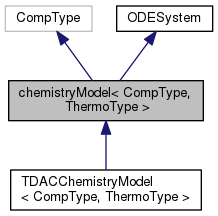[legend]
Collaboration diagram for chemistryModel< CompType, ThermoType >: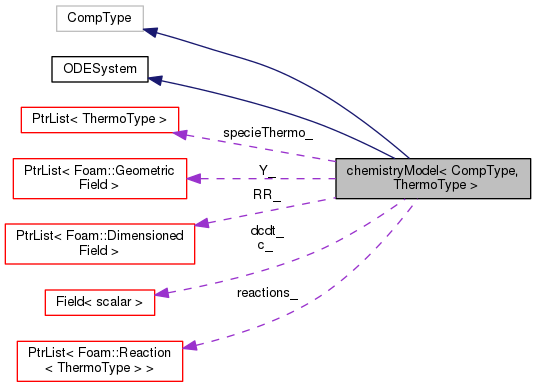[legend]

## Public Member Functions

TypeName ("chemistryModel")
Runtime type information. More...

chemistryModel (const fvMesh &mesh, const word &phaseName)
Construct from mesh. More...

virtual ~chemistryModel ()
Destructor. More...

const PtrList< Reaction< ThermoType > > & reactions () const
The reactions. More...

const PtrList< ThermoType > & specieThermo () const
Thermodynamic data of the species. More...

virtual label nSpecie () const
The number of species. More...

virtual label nReaction () const
The number of reactions. More...

scalar Treact () const
Temperature below which the reaction rates are assumed 0. More...

scalar & Treact ()
Temperature below which the reaction rates are assumed 0. More...

virtual void omega (const scalarField &c, const scalar T, const scalar p, scalarField &dcdt) const
dc/dt = omega, rate of change in concentration, for each species More...

virtual scalar omega (const Reaction< ThermoType > &r, const scalarField &c, const scalar T, const scalar p, scalar &pf, scalar &cf, label &lRef, scalar &pr, scalar &cr, label &rRef) const
Return the reaction rate for reaction r and the reference. More...

virtual scalar omegaI (label iReaction, const scalarField &c, const scalar T, const scalar p, scalar &pf, scalar &cf, label &lRef, scalar &pr, scalar &cr, label &rRef) const
Return the reaction rate for iReaction and the reference. More...

virtual void calculate ()
Calculates the reaction rates. More...

const volScalarField::InternalRR (const label i) const
Return const access to the chemical source terms for specie, i. More...

virtual volScalarField::InternalRR (const label i)
Return non const access to chemical source terms [kg/m3/s]. More...

virtual tmp< volScalarField::InternalcalculateRR (const label reactionI, const label speciei) const
Return reaction rate of the speciei in reactionI. More...

virtual scalar solve (const scalar deltaT)
Solve the reaction system for the given time step. More...

virtual scalar solve (const scalarField &deltaT)
Solve the reaction system for the given time step. More...

virtual tmp< volScalarFieldtc () const
Return the chemical time scale. More...

virtual tmp< volScalarFieldQdot () const
Return the heat release rate [kg/m/s3]. More...

virtual label nEqns () const
Number of ODE's to solve. More...

virtual void derivatives (const scalar t, const scalarField &c, scalarField &dcdt) const
Calculate the derivatives in dydx. More...

virtual void jacobian (const scalar t, const scalarField &c, scalarField &dcdt, scalarSquareMatrix &dfdc) const
Calculate the Jacobian of the system. More...

virtual void solve (scalarField &c, scalar &T, scalar &p, scalar &deltaT, scalar &subDeltaT) const =0

template<class DeltaTType >
Foam::scalar solve (const DeltaTType &deltaT)Public Member Functions inherited from ODESystem
ODESystem ()
Construct null. More...

virtual ~ODESystem ()
Destructor. More...

## Protected Types

typedef ThermoType thermoType

## Protected Member Functions

PtrList< volScalarField::Internal > & RR ()
Write access to chemical source terms. More...

## Protected Attributes

PtrList< volScalarField > & Y_
Reference to the field of specie mass fractions. More...

const PtrList< Reaction< ThermoType > > & reactions_
Reactions. More...

const PtrList< ThermoType > & specieThermo_
Thermodynamic data of the species. More...

label nSpecie_
Number of species. More...

label nReaction_
Number of reactions. More...

scalar Treact_
Temperature below which the reaction rates are assumed 0. More...

PtrList< volScalarField::InternalRR_
List of reaction rate per specie [kg/m3/s]. More...

scalarField c_
Temporary concentration field. More...

scalarField dcdt_
Temporary rate-of-change of concentration field. More...

## Detailed Description

### template<class CompType, class ThermoType> class Foam::chemistryModel< CompType, ThermoType >

Extends base chemistry model by adding a thermo package, and ODE functions. Introduces chemistry equation system and evaluation of chemical source terms.

Source files

Definition at line 58 of file chemistryModel.H.

## ◆ thermoType

 typedef ThermoType thermoType
protected

Definition at line 79 of file chemistryModel.H.

## ◆ chemistryModel()

 chemistryModel ( const fvMesh & mesh, const word & phaseName )

Construct from mesh.

Definition at line 35 of file chemistryModel.C.

Here is the call graph for this function: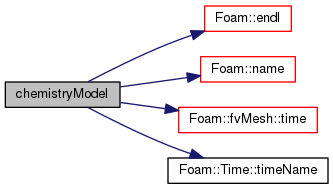## ◆ ~chemistryModel()

 ~chemistryModel ( )
virtual

Destructor.

Definition at line 90 of file chemistryModel.C.

Here is the call graph for this function: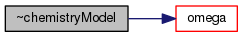## ◆ RR() [1/3]

 Foam::PtrList< Foam::DimensionedField< Foam::scalar, Foam::volMesh > > & RR ( )
inlineprotected

Write access to chemical source terms.

(e.g. for multi-chemistry model)

Definition at line 41 of file chemistryModelI.H.

Here is the caller graph for this function: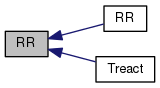## ◆ TypeName()

 TypeName ( "chemistryModel< CompType, ThermoType >" )

Runtime type information.

## ◆ reactions()

 const Foam::PtrList< Foam::Reaction< ThermoType > > & reactions ( ) const
inline

The reactions.

Definition at line 49 of file chemistryModelI.H.

## ◆ specieThermo()

 const Foam::PtrList< ThermoType > & specieThermo ( ) const
inline

Thermodynamic data of the species.

Definition at line 57 of file chemistryModelI.H.

## ◆ nSpecie()

 Foam::label nSpecie ( ) const
inlinevirtual

The number of species.

Definition at line 65 of file chemistryModelI.H.

Here is the caller graph for this function: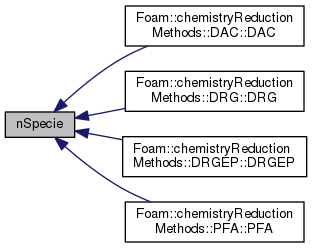## ◆ nReaction()

 Foam::label nReaction ( ) const
inlinevirtual

The number of reactions.

Definition at line 73 of file chemistryModelI.H.

## ◆ Treact() [1/2]

 Foam::scalar Treact ( ) const
inline

Temperature below which the reaction rates are assumed 0.

Definition at line 81 of file chemistryModelI.H.

## ◆ Treact() [2/2]

 Foam::scalar & Treact ( )
inline

Temperature below which the reaction rates are assumed 0.

Definition at line 89 of file chemistryModelI.H.

References chemistryModel< CompType, ThermoType >::RR().

Here is the call graph for this function: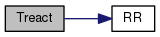## ◆ omega() [1/2]

 void omega ( const scalarField & c, const scalar T, const scalar p, scalarField & dcdt ) const
virtual

dc/dt = omega, rate of change in concentration, for each species

Reimplemented in TDACChemistryModel< CompType, ThermoType >.

Definition at line 98 of file chemistryModel.C.

Here is the call graph for this function: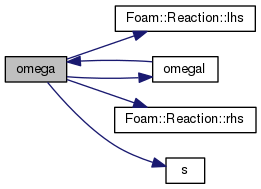Here is the caller graph for this function: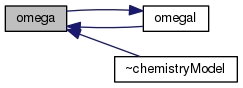## ◆ omega() [2/2]

 Foam::scalar omega ( const Reaction< ThermoType > & r, const scalarField & c, const scalar T, const scalar p, scalar & pf, scalar & cf, label & lRef, scalar & pr, scalar & cr, label & rRef ) const
virtual

Return the reaction rate for reaction r and the reference.

species and charateristic times

Reimplemented in TDACChemistryModel< CompType, ThermoType >.

Definition at line 159 of file chemistryModel.C.

Here is the call graph for this function: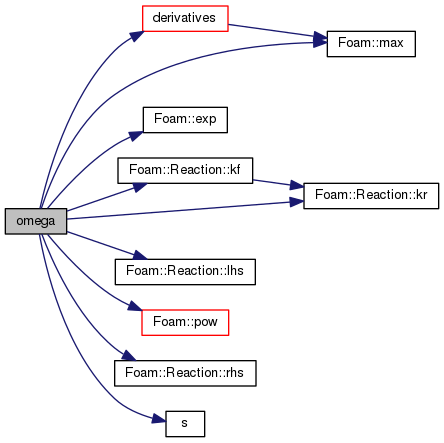## ◆ omegaI()

 Foam::scalar omegaI ( label iReaction, const scalarField & c, const scalar T, const scalar p, scalar & pf, scalar & cf, label & lRef, scalar & pr, scalar & cr, label & rRef ) const
virtual

Return the reaction rate for iReaction and the reference.

species and charateristic times

Definition at line 138 of file chemistryModel.C.

References chemistryModel< CompType, ThermoType >::omega(), and R.

Referenced by chemistryModel< CompType, ThermoType >::omega().

Here is the call graph for this function: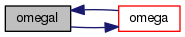Here is the caller graph for this function: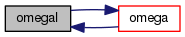## ◆ calculate()

 void calculate ( )
virtual

Calculates the reaction rates.

Definition at line 645 of file chemistryModel.C.

References forAll, Foam::constant::mathematical::pi(), rho, thermo, and trho.

Here is the call graph for this function: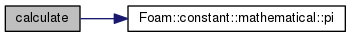## ◆ RR() [2/3]

 const Foam::DimensionedField< Foam::scalar, Foam::volMesh > & RR ( const label i ) const
inline

Return const access to the chemical source terms for specie, i.

Definition at line 98 of file chemistryModelI.H.

References chemistryModel< CompType, ThermoType >::RR().

Here is the call graph for this function: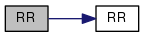## ◆ RR() [3/3]

 Foam::DimensionedField< Foam::scalar, Foam::volMesh > & RR ( const label i )
virtual

Return non const access to chemical source terms [kg/m3/s].

Definition at line 108 of file chemistryModelI.H.

## ◆ calculateRR()

 Foam::tmp< Foam::DimensionedField< Foam::scalar, Foam::volMesh > > calculateRR ( const label reactionI, const label speciei ) const
virtual

Return reaction rate of the speciei in reactionI.

Definition at line 578 of file chemistryModel.C.

Referenced by chemistryModel< CompType, ThermoType >::Qdot().

Here is the call graph for this function: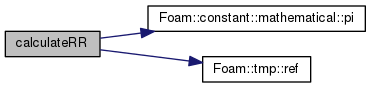Here is the caller graph for this function: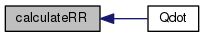## ◆ solve() [1/4]

 Foam::scalar solve ( const scalar deltaT )
virtual

Solve the reaction system for the given time step.

and return the characteristic time

Reimplemented in TDACChemistryModel< CompType, ThermoType >.

Definition at line 753 of file chemistryModel.C.

References Foam::min(), and solve().

Here is the call graph for this function: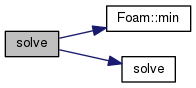## ◆ solve() [2/4]

 Foam::scalar solve ( const scalarField & deltaT )
virtual

Solve the reaction system for the given time step.

and return the characteristic time

Reimplemented in TDACChemistryModel< CompType, ThermoType >.

Definition at line 768 of file chemistryModel.C.

## ◆ tc()

 Foam::tmp< Foam::volScalarField > tc ( ) const
virtual

Return the chemical time scale.

Definition at line 463 of file chemistryModel.C.

Here is the call graph for this function: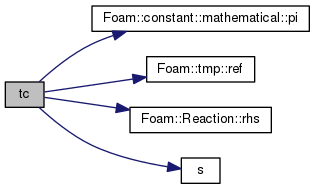## ◆ Qdot()

 Foam::tmp< Foam::volScalarField > Qdot ( ) const
virtual

Return the heat release rate [kg/m/s3].

Definition at line 537 of file chemistryModel.C.

Here is the call graph for this function: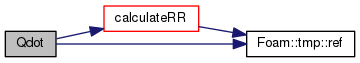## ◆ nEqns()

 Foam::label nEqns ( ) const
inlinevirtual

Number of ODE's to solve.

Implements ODESystem.

Definition at line 32 of file chemistryModelI.H.

## ◆ derivatives()

 void derivatives ( const scalar x, const scalarField & y, scalarField & dydx ) const
virtual

Calculate the derivatives in dydx.

Implements ODESystem.

Reimplemented in TDACChemistryModel< CompType, ThermoType >.

Definition at line 271 of file chemistryModel.C.

References cp, chemistryModel< CompType, ThermoType >::jacobian(), Foam::max(), and rho.

Referenced by chemistryModel< CompType, ThermoType >::omega().

Here is the call graph for this function: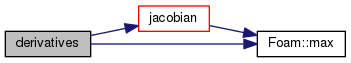Here is the caller graph for this function: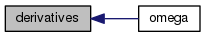## ◆ jacobian()

 void jacobian ( const scalar x, const scalarField & y, scalarField & dfdx, scalarSquareMatrix & dfdy ) const
virtual

Calculate the Jacobian of the system.

Need by the stiff-system solvers

Implements ODESystem.

Reimplemented in TDACChemistryModel< CompType, ThermoType >.

Definition at line 321 of file chemistryModel.C.

Referenced by chemistryModel< CompType, ThermoType >::derivatives().

Here is the call graph for this function: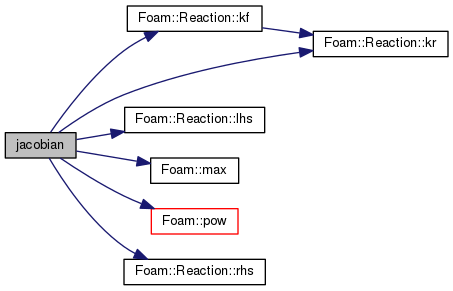Here is the caller graph for this function: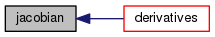## ◆ solve() [3/4]

 virtual void solve ( scalarField & c, scalar & T, scalar & p, scalar & deltaT, scalar & subDeltaT ) const
pure virtual

## ◆ solve() [4/4]

 Foam::scalar solve ( const DeltaTType & deltaT )

Definition at line 683 of file chemistryModel.C.

References correct, forAll, Foam::min(), Foam::constant::mathematical::pi(), rho, solve(), thermo, and trho.

Here is the call graph for this function: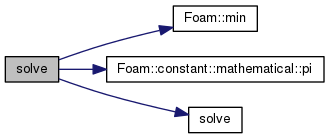## ◆ Y_

 PtrList& Y_
protected

Reference to the field of specie mass fractions.

Definition at line 84 of file chemistryModel.H.

## ◆ reactions_

 const PtrList >& reactions_
protected

Reactions.

Definition at line 87 of file chemistryModel.H.

## ◆ specieThermo_

 const PtrList& specieThermo_
protected

Thermodynamic data of the species.

Definition at line 90 of file chemistryModel.H.

## ◆ nSpecie_

 label nSpecie_
protected

Number of species.

Definition at line 93 of file chemistryModel.H.

## ◆ nReaction_

 label nReaction_
protected

Number of reactions.

Definition at line 96 of file chemistryModel.H.

## ◆ Treact_

 scalar Treact_
protected

Temperature below which the reaction rates are assumed 0.

Definition at line 99 of file chemistryModel.H.

## ◆ RR_

 protected

List of reaction rate per specie [kg/m3/s].

Definition at line 102 of file chemistryModel.H.

## ◆ c_

 scalarField c_
mutableprotected

Temporary concentration field.

Definition at line 105 of file chemistryModel.H.

## ◆ dcdt_

 scalarField dcdt_
mutableprotected

Temporary rate-of-change of concentration field.

Definition at line 108 of file chemistryModel.H.

The documentation for this class was generated from the following files:
• src/thermophysicalModels/chemistryModel/chemistryModel/chemistryModel/chemistryModel.H
• src/thermophysicalModels/chemistryModel/chemistryModel/chemistryModel/chemistryModel.C
• src/thermophysicalModels/chemistryModel/chemistryModel/chemistryModel/chemistryModelI.H